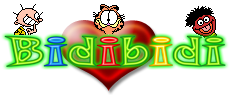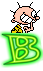Play, Have Fun, Spend Time. Send Score - Print Record. Be the First! We have been with you for 16 years

You Must Login for Send Records and Play Unlimited!You will play for about 4 minutes. Average Score: 642,073

#I am the plane, you are the planeLet's see who will shoot who. Play with the mouse, the left mouse button shoots. we wish you good luck.

Controls

2.107played times 6.193views

The Bests - I am the plane, you are the plane
All Times

9.731,000burakcan76000

This Year
-

This Month
-

Today
-

Rewards & Costs - I am the plane, you are the plane
All Times record
+25
This Year record
+22
This Month record
+7
Today record
+0.25
Playing Award:
+0.01
Game Cost
-0.00

Comments Last 5 - I am the plane, you are the planekarahanli2 17th Aug 2008 , 16:51

arkadaşlar bi kaç gündür geliyorum bakıyorum hiç msj felan yok iştahım kaçıyo geri oynamadan gidiyorum:) rekorklar kırılmak içi3n var :) bu arada sıla seni azimli ve hırslı gördüm bol şanslar:):)apache 26th Jul 2008 , 16:59

:):):):):):):):):):):):):):):):):):):):):):):):):):):):):):):):):):):):):):):):):):):):):):):):):):):):):):):):):):):):):):):):):):):):):):):):):):):):):):):):):):):):):):):):):):):):):):):):):):):):):):):):):):):):):):):):):):):):):):):):):):):):):):)